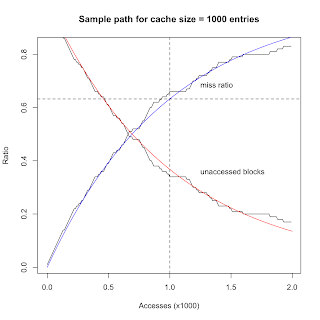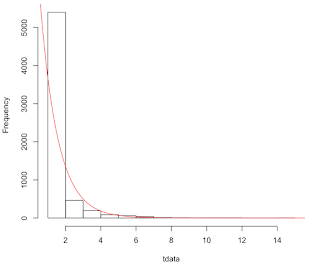## Wednesday, May 15, 2013

### Exponential Cache Behavior

Guerrilla alumnus Gary Little observed certain fixed-point behavior in simulations where disk IO blocks are updated randomly in a fixed size cache. For his python simulation with 10 million entries (corresponding to an allocation of about 400 MB of memory) the following results were obtained:
• Hit ratio (i.e., occupied) = 0.3676748
• Miss ratio (i.e., inserts) = 0.6323252

In other words, only 63.23% of the blocks will ever end up inserted into the cache, irrespective of the actual cache size. Gary found that WolframAlpha suggests the relation: \begin{equation} \dfrac{e-1}{e} \approx 0.6321 \label{eq:walpha} \end{equation} where $e = \exp(1)$. The question remains, however, where does that magic fraction come from?

To answer that question, I'm going to apply my VAMOOS technique: Visualize, Analyze, Modelize, Over and Over, until Satisfied to various cache simulation results.

### Visualize

First, let's plot the sample paths for the number of cache hits and misses for a simulation of 1000 cache accesses. That's long enough to get a general feel for what is going on. The posted python code was run for much longer, but that was mostly to improve the accuracy of the observed miss ratio against eqn. $\eqref{eq:walpha}$Figure 1. Cache sample paths

We see immediately from Fig. 1 that the miss count rises in a slightly concave fashion to a termination value of 646 (near the intersection of the dashed gridlines at 632.33), and the hit count rises in a slightly convex fashion to a termination value of 354. The respective hit and miss ratios for 1000 accesses are 0.646 and 0.354. The first of these is close to the magic number. Similar results are found for repeated simulations using a different number of total accesses. This is what Gary observed numerically, but now we can visualize the actual evolution toward those fixed points.

### Analyze

Next, let's focus on the upper curve in Fig. 1, since it terminates at 63.23% of the cache blocks—the magic number. To examine this aspect more closely, I rewrote the python code in the R language to make it a little more efficient.


csize <- 1e3
cache <- rep.int(0, times=csize)
b     <- round(runif(csize, min=1, max=csize))
miss  <- 0

for(i in 1:csize * 2) {     # 2x cache size
if(cache[b[i]] == 0) {  # read
cache[b[i]] <- 1    # write
miss <- miss + 1    # faster than %+=% in operators pkg
}
}


More importantly, I ran the R code for twice as many accesses as the original python code. An example result is shown in Fig. 2.Figure 2. Cache-miss sample path compared with eqn. $\eqref{eq:walpha}$ (blue curve)

Notice that the sample path continues its concave trajectory beyond the claimed termination point at the intersection of the dashed gridlines. In fact, it approaches 100% cache inserts, asymptotically. Even without doing any fancy regression fitting, I can see (visually) that this sample path is tracking the (blue) curve given by \begin{equation} 1 - e^{-\lambda t} \label{eq:expCDF} \end{equation} where t is the simulation time-step corresponding to the number of cache accesses and λ is a growth-rate parameter. But eqn. $\eqref{eq:expCDF}$ is the CDF (cumulative distribution function) for an exponentially distributed random variable with mean value 1/λ. I can easily verify this conclusion using R:

> qexp(0.6323252, rate=1)
 1.000556

The 63.23 quantile (horizontal dashed line) of an exponential distribution with rate parameter λ = 1 is the mean 1/λ = 1 on the x-axis of Fig. 2. (cf. Fig. 1 in "Adding Percentiles to PDQ")

That also tells us that 63.23% is not a magical fraction, but merely the last value of the sample path if the termination value of the simulation for-loop is chosen equal to the cache size.

In Fig. 2, I rescaled the number of accesses on x-axis by the cache size (1000 blocks). Therefore, the simulation reaches the cache size at the renormalized time t = 1, but continues for twice that number of time-steps before the for-loop terminates (see R code above). Since λ = 1, at the renormalized time t = 1.0 eqn. $\eqref{eq:expCDF}$ is identical to eqn. $\eqref{eq:walpha}$.

That explains the mysterious WolframAlpha correspondence.

### Modelize

So far, we've seen that the connection with the exponential distribution appears to be real and that the mysterious 63.23% miss fraction is nothing more than a coincidence due to arbitrarily terminating the for-loop at the cache size. But all this still begs the question, what process is actually being modeled in the original python simulation?

Fig. 3 compares the exponential miss-ratio sample path together with the corresponding ratio of the remaining unoccupied slots in the cache. Clearly, this sample path decreases over time and will approach zero capacity eventually.Figure 3. Exponential CDF (blue curve) and PDF (red curve)

The red curve corresponds to the function that is the complement of eqn. $\eqref{eq:expCDF}$, viz., \begin{equation} e^{-\lambda t} \label{eq:expdecay} \end{equation} with λ = 1, as before. Equation \eqref{eq:expdecay} simply characterizes the exponential decay of the cache capacity, starting with 100% available cache slots. Quite literally, this means that Gary's original simulation is the analog of a box of radioactive atoms whose nuclei are decaying with a half-life given by \begin{equation} t_{1/2} = \dfrac{\ln(2)}{\lambda} \end{equation} Since λ = 1 the 0.50 fraction (on the y-axis) or, by analogy, 50% of the box of atoms will have decayed by time

> log(2)
 0.6931472

in the normalized time units of Fig. 2 or 69.31 time steps. That's also where the blue and red curves intersect in Fig. 3.
For those unfamiliar, this is what weak radioactive decay events sound like on a Geiger counter. That's also the sound of Gary's cache being updated.
In other words, each '0' in the initialized cache is analogous to the undecayed nucleus of an imaginary atom. During the simulation, an atom is selected at random and decayed to a '1' state, if it hadn't already decayed. The more atoms that have already decayed, the longer it will take to find an as yet undecayed atom. See Fig. 4.Figure 4. Histogram of the periods between cache updates

To check this idea, I rewrote my simulation in R to count the time periods between each nuclear decay.


csize <- 1e6
cache <- rep.int(0, times=csize)
b     <- round(runif(csize, min=1, max=csize))
tprev <- 0
tdata <- NULL

for(i in 1:csize) {
if(cache[b[i]] == 0) {    # read  = undecayed "nucleus"
cache[b[i]] <- 1      # write = decay the "nucleus"
tdelta <- (i - tprev) # period since last decay
tprev  <- i
tdata  <- append(tdata, tdelta)
}
}

sample.mean <- sum(tdata) / csize
sample.sdev <- sqrt(sum((tdata-sample.mean)^2) / (csize-1))

cat(sprintf("Iterations:  %s\n", prettyNum(i, big.mark = ",")))
print("Decay period statistics:-")
cat(sprintf("Mean: %f\n", sample.mean))
cat(sprintf("SDev: %f\n", sample.sdev))
cat(sprintf("CoV : %f\n", sample.sdev / sample.mean))

Example outputs for 100 thousand and 1 million time-steps look like this:
> system.time(source(".../cache-stats.r"))
Iterations:  100,000
 "Decay period statistics:-"
Mean: 0.999990
SDev: 1.041080
CoV : 1.041091

> system.time(source(".../cache-stats.r"))
Iterations:  1,000,000
 "Decay period statistics:-"
Mean: 0.999997
SDev: 1.034501
CoV : 1.034505


Since the coefficient of variation CoV = 1, it's clear that we are indeed looking at exponentially distributed decay periods. Note that we can't use the R functions mean() and sd() because tdata in the simulation is less than the total number of time steps. Moreover, since exponentially distributed periods between events are produced by a Poisson source, it appears that Gary's simulation is actually a model of a Poisson stochastic process.

### Satisfied?

OK, I think I'm satisfied that I understand what Gary has simulated. It appears to be a Poisson generator of insert events (a point process) and therefore exhibits exponentially distributed periods between inserts. This means it also models the exponential interarrival times into a queueing facility and maybe I can use this in my Guerrilla classes to avoid getting into too much probability theory. Normally, I show a movie of cars queueing up at a closed railway gate.

On the other hand, I'm not sure if Gary's model represents what the real disk cache does. What still puzzles me is that everything I know about cache behavior (which isn't that much) tells me they tend to exhibit fractal-like power law scaling, not exponential scaling. But that will largely be determined by the particular cache updating protocol. Maybe there are exceptions and this is one of them.